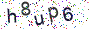## Reply to Re: image_auto_rotate is very slow

Re: image_auto_rotate is very slow new!
by Dominik, 1 year, 9 months ago
Hi Colin,

I have some update for you.
Haven't heard of you for a long time, so I did some code changes myself regarding the veeeery slow image_auto_rotate and auto_flip functions. (In fact sadly these aren't real functions but simple if-statements, if these would have been functions/methods I could have easily extended your class and create overrides methods...)

Here is the code I changed:
In your \$auto_flip statement you need to replace this (Old):

```for (\$x = 0; \$x < \$this->image_src_x; \$x++) {
for (\$y = 0; \$y < \$this->image_src_y; \$y++){
if (strpos(\$auto_flip, 'v') !== false) {
imagecopy(\$tmp, \$image_dst, \$this->image_src_x - \$x - 1, \$y, \$x, \$y, 1, 1);
} else {
imagecopy(\$tmp, \$image_dst, \$x, \$this->image_src_y - \$y - 1, \$x, \$y, 1, 1);
}
}
}```

with this (New):

```// Copy the image
imagecopy(\$tmp, \$image_dst, 0, 0, 0, 0, \$this->image_src_x, \$this->image_src_y);

// Flip the image
if (!imageflip(\$tmp, (\$auto_flip == 'h' ? IMG_FLIP_HORIZONTAL : IMG_FLIP_VERTICAL)))
{
throw new \LogicException('Unable to flip the image.');
}```

And in your \$auto_rotate statement you need to replace this (Old):

```if (\$auto_rotate == 90 || \$auto_rotate == 270) {
\$tmp = \$this->imagecreatenew(\$this->image_src_y, \$this->image_src_x);
} else {
\$tmp = \$this->imagecreatenew(\$this->image_src_x, \$this->image_src_y);
}
\$this->log .= '- auto-rotate image : ' . \$auto_rotate . '';
for (\$x = 0; \$x < \$this->image_src_x; \$x++) {
for (\$y = 0; \$y < \$this->image_src_y; \$y++){
if (\$auto_rotate == 90) {
imagecopy(\$tmp, \$image_dst, \$y, \$x, \$x, \$this->image_src_y - \$y - 1, 1, 1);
} else if (\$auto_rotate == 180) {
imagecopy(\$tmp, \$image_dst, \$x, \$y, \$this->image_src_x - \$x - 1, \$this->image_src_y - \$y - 1, 1, 1);
} else if (\$auto_rotate == 270) {
imagecopy(\$tmp, \$image_dst, \$y, \$x, \$this->image_src_x - \$x - 1, \$y, 1, 1);
} else {
imagecopy(\$tmp, \$image_dst, \$x, \$y, \$x, \$y, 1, 1);
}
}
}```

with this (New):

```\$tmp = \$this->imagecreatenew(\$this->image_src_x, \$this->image_src_y);
\$this->log .= '- auto-rotate image : ' . \$auto_rotate . '';

// Copy the image
imagecopy(\$tmp, \$image_dst, 0, 0, 0, 0, \$this->image_src_x, \$this->image_src_y);

// Rotate the image
\$tmp = imagerotate(\$tmp, \$auto_rotate, 0);```

To make it rotate the images correctly you also have to change your exif-orientation cases to this (New):

```switch(\$orientation) {
case 1:
\$this->log .= '- EXIF orientation = 1 : default';
break;
case 2:
\$auto_flip = 'h';
\$this->log .= '- EXIF orientation = 2 : horizontal flip';
break;
case 3:
\$auto_rotate = 180;
\$this->log .= '- EXIF orientation = 3 : 180 rotate';
break;
case 4:
\$auto_flip = 'h';
\$auto_rotate = 180;
\$this->log .= '- EXIF orientation = 4 : horizontal flip + 180 rotate';
break;
case 5:
\$auto_flip = 'h';
\$auto_rotate = 90;
\$this->log .= '- EXIF orientation = 5 : horizontal flip + 90 rotate left';
break;
case 6:
\$auto_rotate = 270;
\$this->log .= '- EXIF orientation = 6 : 270 rotate left';
break;
case 7:
\$auto_flip = 'h';
\$auto_rotate = 270;
\$this->log .= '- EXIF orientation = 7 : horizontal flip + 270 rotate left';
break;
case 8:
\$auto_rotate = 90;
\$this->log .= '- EXIF orientation = 8 : 90 rotate left';
break;
default:
\$this->log .= '- EXIF orientation = '.\$orientation.' : unknown';
break;
}```

Hope you can use it!
 Name * Email Title * Text *Enter the code displayed on the image: Click on the image to generate another one if it is hard to read it. The case is important